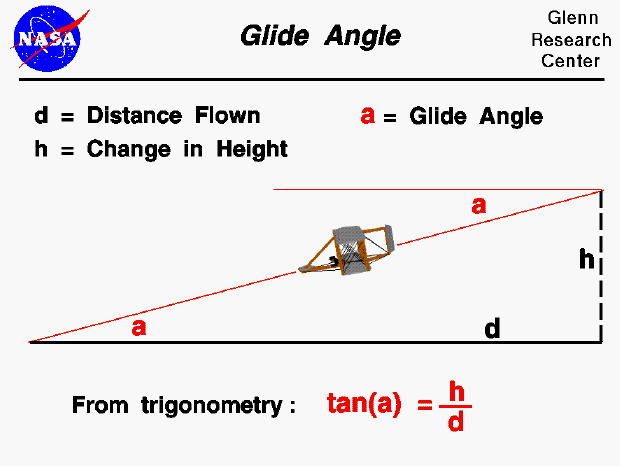A glider is a special kind of aircraft that has no engine. Paper airplanes are the most obvious example, but gliders come a wide range of sizes. Toy gliders, made of balsa wood or styrofoam, are an excellent way for students to study the basics of aerodynamics. The Wright brothers perfected the design of the first airplane and gained piloting experience through a series of glider flights. These aircraft were similar to modern hang-gliders and were launched by running off a hill.

If a glider is in a steady (constant velocity and no acceleration) descent, it loses altitude as it travels. The glider's flight path is a simple straight line, shown as the inclined red line in the figure. The flight path intersects the ground at an angle "a" called the glide angle. If we know the distance flown and the altitude change, we can calculate the glide angle using trigonometry. The tangent (tan) of the glide angle (a) is equal to the change in height (h) divided by the distance flown (d).

If the glider is flown at a specified glide angle, the trigonometric equation can be solved to determine how far the glider can fly for a given change in altitude. Notice that if the glide angle is small, the aircraft can fly a long distance for a small change in altitude. Conversely, if the glide is large it can travel only a short distance for a given change in altitude. We can think of the glide angle as a measure of the flying efficiency of the glider. On another page, we will show that the glide angle is inversely related to the lift to drag ratio. The smaller the glide angle, the higher the lift to drag ratio.

Guided Tours